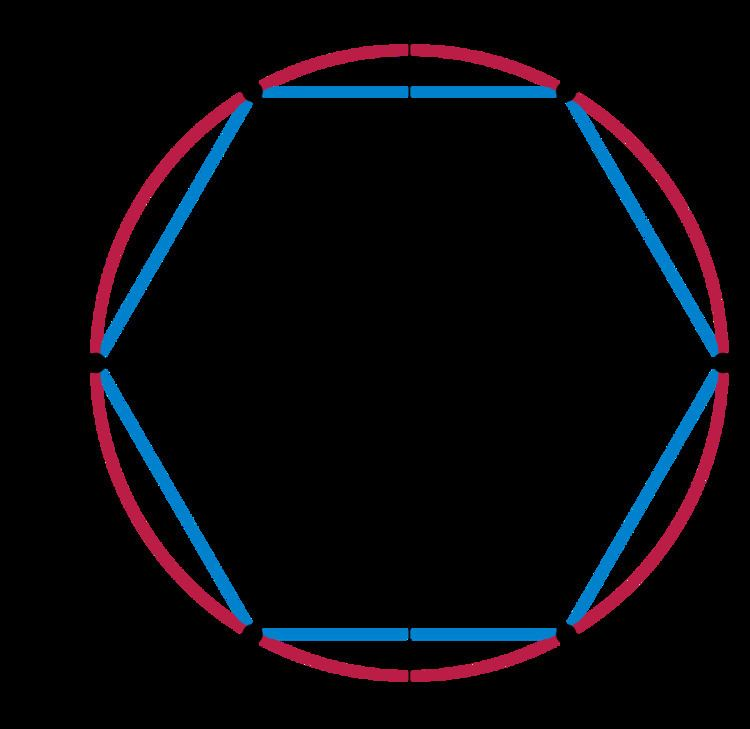# Mathieu group M23

Updated on
Covid-19In the area of modern algebra known as group theory, the Mathieu group M23 is a sporadic simple group of order

## Contents

27 · 32 ··· 11 · 23 = 10200960 ≈ 1×107.

## History and properties

M23 is one of the 26 sporadic groups and was introduced by Mathieu (1861, 1873). It is a 4-fold transitive permutation group on 23 objects. The Schur multiplier and the outer automorphism group are both trivial.

Milgram (2000) calculated the integral cohomology, and showed in particular that M23 has the unusual property that the first 4 integral homology groups all vanish.

The inverse Galois problem seems to be unsolved for M23. In other words, no polynomial in Z[x] seems to be known to have M23 as its Galois group. The inverse Galois problem is solved for all other sporadic simple groups.

## Representations

M23 is the point stabilizer of the action of the Mathieu group M24 on 24 points, giving it a 4-transitive permutation representation on 23 points with point stabilizer the Mathieu group M22.

M23 has 2 different rank 3 actions on 253 points. One is the action on unordered pairs with orbit sizes 1+42+210 and point stabilizer M21.2, and the other is the action on heptads with orbit sizes 1+112+140 and point stabilizer 24.A7.

The integral representation corresponding to the permutation action on 23 points decomposes into the trivial representation and a 22-dimensional representation. The 23 dimensional representation gives an irreducible representation over any field of characteristic not 2 or 23.

Over the field of order 2, it has 2 11-dimensional representations, the restrictions of the corresponding representations of the Mathieu group M24.

## Maximal subgroups

There are 7 conjugacy classes of maximal subgroups of M23 as follows:

• M22, order 443520
• PSL(3,4):2, order 40320, orbits of 21 and 2
• 24:A7, order 40320, orbits of 7 and 16
• Stabilizer of W23 block
• A8, order 20160, orbits of 8 and 15
• M11, order 7920, orbits of 11 and 12
• (24:A5):S3 or M20:S3, order 5760, orbits of 3 and 20 (5 blocks of 4)
• One-point stabilizer of the sextet group
• 23:11, order 253, simply transitive
• ## References

Similar Topics
Thenavattu
David Bonneville
Patrick Préjean
Topics